# Common Core: High School - Geometry : Congruence

## Example Questions

### Example Question #71 : Congruence

Jenny has drawn a rectangle in the upper left hand corner of a piece of paper. If she folds the paper in half and traces the rectangle on the other half of the paper, what type of rigid motion has occurred?

None of the other answers

Expansion

isometry

Reflection

Rotation

Reflection

Explanation:

This situation describes a real life application of geometry where the coordinate grid can be seen as the piece of paper. Since Jenny is tracing the rectangle after the paper is folded it can be seen that the fold is an axis and the new rectangle is congruent to the original one. Therefore, the rigid motion describing this situation is a reflection.

### Example Question #72 : Congruence

Jill and Jane sit next to each other in math class. Jill has a sheet of paper and Jane asks her for half of it to take notes on because she left her notebook in the locker. If Jill cuts the sheet of paper in half, has a rigid motion occurred?

No

Yes

No

Explanation:

Recall that a rigid motion is that that preserves the distances between points within the object while undergoing a motion in the plane. This is also called an isometry, rigid transformations, or congruence transformations and there are four different types. The piece of paper is the object in this situation. Since the paper is cut in half, it does not preserve the shape of the object and thus, is not a rigid motion.

Therefore, the answer is "No".

### Example Question #1 : Definition Of Congruency For Triangles: Ccss.Math.Content.Hsg Co.B.7

What must be true if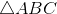has had a rigid motion applied to it to result in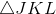?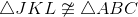Only two angles from each triangle are congruent

None of the other answers

Only corresponding angles are congruent

Both corresponding angles and sides are congruent

Both corresponding angles and sides are congruent

Explanation:

Recall that a rigid motion preserves the distance from points within a shape in the plane. Therefore, ifhas had a rigid motion applied to it to result inthat means that all corresponding sides and angles of these two triangle are congruent.

Therefore, the statement "both corresponding angles and sides are congruent" is the correct solution to this particular question.

### Example Question #2 : Definition Of Congruency For Triangles: Ccss.Math.Content.Hsg Co.B.7

Determine whether the statement is true or false:

Two triangles that are congruent have corresponding points that are separated five spaces. These two triangles describe a rigid motion.

False

True

True

Explanation:

Recall that a rigid motion preserves the distance from points within a shape in the plane. Since the statement clearly states that the two triangles are congruent, that means that the distances between the points within the shape are preserved. Also, since the corresponding points between the two triangles are separated by five spaces this is describing a translation which when combined with the preserved shape, describes a rigid motion. Therefore, the statement is true.

### Example Question #2 : Definition Of Congruency For Triangles: Ccss.Math.Content.Hsg Co.B.7

When a rigid motion is done onto A, C is the resulting image. Are triangles A and C congruent?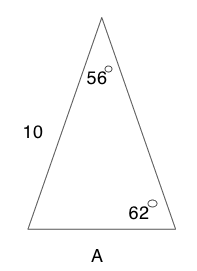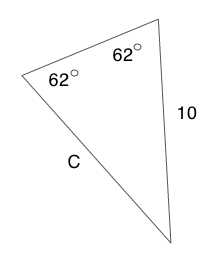No

Yes

Yes

Explanation:

Recall that a rigid motion preserves the distance from points within a shape in the plane. It appears that the rigid motion was a rotation. The third angle can be identified by subtracting the two known angles from 180 degrees.

Triangle A: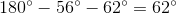Triangle C: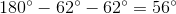Since the side between two angles on triangle C is congruent to the side between those same two angles on triangle A, the two triangles are congruent.

### Example Question #3 : Definition Of Congruency For Triangles: Ccss.Math.Content.Hsg Co.B.7

Determine whether the statement is true or false.

An equilateral triangleis rotated counter clockwise and transformed into an isosceles triangle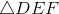is congruent to.

False

True

False

Explanation:

To determine whether this statement is true or false, first recall what it means to be "congruent". Congruent means equal or in more mathematical terms, both corresponding angles and sides of the two triangles are congruent.

The statement says thatis an equilateral triangle which means all sides and angles are the same. After the triangle goes through a transformation, it becomes an isosceles triangle. To be isosceles means that two of the sides and angles of the triangle are the same. Since, not all of the angles are the same init is not congruent to.

Therefore, the statement, "is congruent to." is false.

### Example Question #1 : Definition Of Congruency For Triangles: Ccss.Math.Content.Hsg Co.B.7

Which of the following geometric theorems cannot prove triangle congruency?

Side, Angle, Side

Hypotenuse, Leg of Right Triangle

Angle, Angle, Angle

Angle, Side, Angle

Side, Side, Side

Angle, Angle, Angle

Explanation:

To determine which theorem cannot prove triangle congruency, first recall what it means to be "congruent". Congruent means equal or in more mathematical terms, both corresponding angles and sides of the two triangles are congruent.

There are five theorems used in geometry to prove whether two triangles are congruent.

1. Side, Side, Side

2. Side, Angle, Side

3. Angle, Side, Angle

4. Angle, Angle, Side

5, Hypotenuse and One Leg from Right Triangle

Of the answers below, Angle, Angle, Angle (AAA) is not among one of the theorems to prove triangle congruency. The reason AAA does not prove triangle congruency because two triangles can have the same angles but have different side lengths thus, the triangles would not be congruent.

### Example Question #4 : Definition Of Congruency For Triangles: Ccss.Math.Content.Hsg Co.B.7

Which theorem can be used to prove triangle congruency between triangle A and C?HL

AAS

AAA

SSS

SAS

AAS

Explanation:

For this particular problem there are two geometric theorems that can prove that the triangles are similar.

The geometric theorems that could be used:

1. Angle, Side, Angle (ASA)

2. Angle, Angle, Side (AAS)

For triangle C the AAS is the most evident to use. Since ASA is not an option in the answer selections, AAS is the correct answer.

### Example Question #5 : Definition Of Congruency For Triangles: Ccss.Math.Content.Hsg Co.B.7

Which theorem can be used to prove triangle congruency between triangle A and C?ASA

SAS

AAA

HL

SSS

ASA

Explanation:

For this particular problem there are two geometric theorems that can prove that the triangles are similar.

The geometric theorems that could be used:

1. Angle, Side, Angle (ASA)

2. Angle, Angle, Side (AAS)

For triangle C the AAS is the most evident to use. Since AAS is not an option in the answer selections, ASA is the correct answer.

### Example Question #6 : Definition Of Congruency For Triangles: Ccss.Math.Content.Hsg Co.B.7

Which theorem can be used to prove triangle congruency between triangle A and B?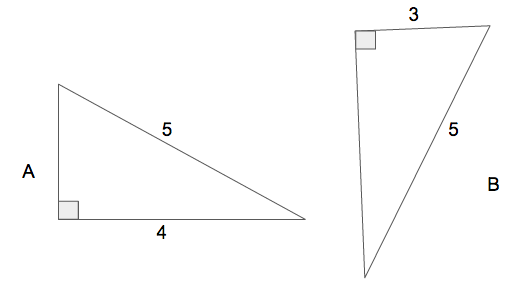SAS

HL

AAS

AAA

ASA

HL

Explanation:

For this particular problem there are two geometric theorems that could potentially be used to prove that the triangles are similar.

The geometric theorems that could be used:

1. Hypotenuse and One Leg of a Right Triangle (HL)

2. Side, Side, Side (SSS)

To use SSS or HL, the Pythagorean theorem will need to be used to calculate the missing side.

For these triangles the HL is the most evident to use and since SSS is not an option in the answer selections, HL is the correct answer.

### All Common Core: High School - Geometry Resources